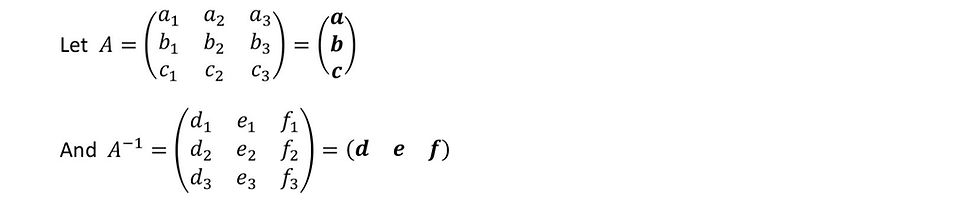top of page
Search
• Martin Scarratt

# An easier way to find the inverse of a 3x3 matrixFinding the inverse of a 3x3 matrix can be a bit of a slog. Not particularly difficult, but there are a number of long and intricate steps that need to be taken before you finally end up with the answer (which is often wrong as during the second step your eyes glazed over with boredom and you wrote a 3 instead of a -3).

The usual method is:

1. Find the determinant

2. Find the matrix of minors

3. Find the matrix of co-factors

4. Transpose

5. Divide by the determinant

This method will work for any square matrix larger than a 2x2 matrix (the 2x2 matrix having its own nice simple way of finding its inverse).

There is a little known quick method for a 3x3 matrix too! All you need to know is how to take the cross product of two vectors.The result of the cross product is a new vector that is perpendicular to both of the vectors that were crossed.

There are reasons for this, but i'm not going to go into it here as it can be found in any maths book that attempts to explain the cross product.

Now, armed with this knowledge I can introduce you to the formula for the 3x3 inverse. But of course, I'm not just going to tell you what it is, I'm going to show you how it comes about too, so put on your thinking cap and we can begin.

Finding the inverse of a 3x3 matrix using the vector (cross) productOur aim then is to find all the elements of this last matrix, i.e. the elements of the three column vectors d, e and f.This we know by the definition of the inverse matrix and by using matrix multiplication (multiplying matrices is really just dotting rows of the first matrix with columns of the second and writing them as a new matrix).And we find we have one of the column vectors that we were looking for (as long as we know what a, b and c are – which we do, they are the row vectors of the matrix we are trying to find the inverse of).

Following the exact same arguments for columns 2 and 3 of gives similar equations for e and f giving us a formula for the inverse of a 3x3 matrix.So there you have it. This is a formula for the inverse of a 3x3 matrix using the cross product. It looks a little scary at first glance but it is actually very easy to use once you are used to working out cross products. The example at the end of this article shows you how quick it really is, but first a couple of observations that can make things even easier:The first result given above is the cyclic property of what is called the scalar triple product (the determinant when talking about matrices). You can find an explanation to this in any good book on vectors (my favourite explanation involves looking at volume of the parallelopiped using a different base each time).

And now, finally, an example of actually using this method to calculate the inverse of a 3x3 matrixWe now have all the results we need, all that remains is to write these vectors as columns and divide by the determinant.If you spend some time thinking about this method carefully you will see that we are actually following the steps of the traditional method but in a quicker, more sophisticated way that actually has a meaning. You can see from the above workings why this works. I have never seen an explanation as to why the traditional method works, although the cross product method can be adapted to provide such an explanation - but why would you want to do that once you know the cross product method?